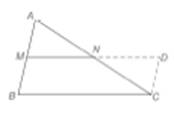Chapter 4.2, Problem 39E### Elementary Geometry for College St...

6th Edition
Daniel C. Alexander + 1 other
ISBN: 9781285195698

#### Solutions

Chapter
Section### Elementary Geometry for College St...

6th Edition
Daniel C. Alexander + 1 other
ISBN: 9781285195698
Textbook Problem
16 views

# Prove that the segment that joins the midpoints of two sides of a triangle has a length equal to one-half the length of the third side.(HINT: In the drawing, M N ¯ is extended to D , a point on C D ¯ . Also, C D ¯ is a parallel to A B ¯ .)To determine

To prove:

The segment that joins the midpoints of two sides of a triangle has a length equal to one-half the length of the third side.

Explanation

Formal proof:

Statement:

The segment that joins the midpoints of two sides of a triangle has a length equal to one-half the length of the third side.

Drawing:

Given:

ABC is a triangle with M and N as the midpoint of the sides AB and AC respectively.

ANCN

AMBM

Prove:

MN=12BC

 PROOF Statement Reasons 1. AM¯≅BM¯AN¯≅CN¯ 1. Given 2. Produce MN to D to meet CD which is parallel to AB. 2. Construct a parallelogram BCDM 3

### Still sussing out bartleby?

Check out a sample textbook solution.

See a sample solution

#### The Solution to Your Study Problems

Bartleby provides explanations to thousands of textbook problems written by our experts, many with advanced degrees!

Get Started

#### 14. At write the equation of the line tangent to

Mathematical Applications for the Management, Life, and Social Sciences

#### The area of the region bounded by , , and r = sec θ is:

Study Guide for Stewart's Single Variable Calculus: Early Transcendentals, 8th

#### Describe the general characteristics of the survey research design.

Research Methods for the Behavioral Sciences (MindTap Course List)